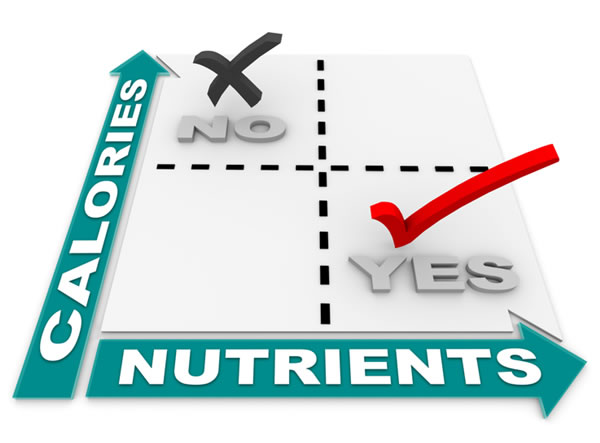Weight loss tips

# What’s the recommended calorie intake for women to lose weight?The recommended calorie intake for women to lose weight depends on the following factors: age, weight, height and activity level. Let’s see how you can calculate your Body Mass Index (BMI), Basic Metabolic Rate (BMR) and the number of calories you should eat per day in order to lose weight.

## Step 1: Calculate your Body Mass Index (BMI)

Depending on your BMI you belong to one of the following categories:

BMI of 18.5 or less –> Underweight
BMI of 18.5 to 24.99–>Normal Weight
BMI of 25 to 29.99 –>Overweight
BMI of 30 to 34.99 –> Obesity (Class 1)
BMI of 35 to 39.99 –> Obesity (Class 2)
BMI of 40 or greater–> Morbid Obesity

This is more of a scientific classification of your weight and does not necessary mean that if you have a normal BMI (18.5 -24.99) you do not need to lose weight. If you want to lose weight or not this depends on how you feel about your body. The BMI calculation is just an indication that is used for medical purposes. To calculate your BMI you need to apply the following equation:

BMI= (weight in pounds * 703) / (height in inches) x (height in inches)

or

BMI= (weight in Kg) / (height in meters) x (height in meters)

## Step 2: Calculate your Basic Metabolic Rate (BMR)

BMR is the amount of calories our body needs to carry out basic body functions such as breathing, digestion, blood circulation etc. It is the minimum amount of calories you spend each day. You can calculate your BMR using the following equation. Please note that the formula below applies for WOMEN only:

BMR = 655 + (4.3 x weight in pounds) + (4.7 x height in inches) – (4.7 x age in years)

Or

BMR = 655.0955 + (9.5634 x weight kg) + (1.8496 x height cm) – (4.6756 x age)

## Step 3: Calculate how many calories you need per day

Once you know your BMR you can calculate how many calories you need per day in order to maintain your current weight. This number is important because if you want to lose weight you need to consume fewer calories than what you need. To calculate your daily calorie requirements you can use the following formula depending on your lifestyle:

If you are sedentary (minimum or no physical activities): BMR + (BMR x 20 %)

If you are lightly active (doing some form of light exercise, e.g. walking): BMR + (BMR x 30%)

If you are moderately active (exercise at least 3 times per week): BMR + (BMR x 40%)

If you are very active (exercise daily): BMR + (BMR x 50%)

If you are extra active (intensive work out on a daily basis): BMR + (BMR x 60%)

The table below shows the average daily calorie requirements for women based on their weight.

 Weight Female 50 kg / 110 pounds 1680 Cal per day 60 kg / 132 pounds 1800 Cal per day 70 kg / 154 pounds 1920 Cal per day 80 kg / 176 pounds 2040 Cal per day 90 kg / 198 pounds 2155 Cal per day 100 kg /240 pounds 2270 Cal per day

### Calorie intake for women to lose weight

Ok, so you have calculated all the above numbers but what do these numbers actually mean? Let’s demonstrate this will a real example.

The basic Data: Woman aged 40, Current Weight: 70Kg, Height 1.60M, Lifestyle: Lightly active (doing office work, household activities and walking for 1 hour per week).

Calculating the BMI

BMI= (weight in Kg) / (height in meters) x (height in meters)

BMI = 70 / (1.6×1.6) = 27.34

A BMI of 27.34 tells us that this woman is in the category of overweight.

Calculating BMR

BMR = 655.0955 + (9.5634 x weight kg) + (1.8496 x height cm) – (4.6756 x age)

BMR = 655.0955 + (9.5634 x 70) + (1.8496 x 160) – (4.6756 x 40) = 1433 Calories

1433 is the number of calories needed by this woman’s body to carry out normal functioning.

Calculating Daily Calorie Needs

For lightly active (doing some form of light exercise, e.g. walking): BMR + (BMR x 30%)

i.e. 1433 + 430 = 1863 Calories

1863 Calories are needed per day for this woman to maintain her current weight of 70Kg. For this particular woman to lose weight she should consume fewer than 1863 calories per day. If her goal is to lose 2 pounds a month then she needs to save 7000 calories per month (1 pound of fat is 3500 calories). So, to be able to save 7000 calories per month she should reduce her calorie intake by 233 calories. This means that the max amount of calories to consume per day in order to lose weight is 1863-233 = 1630. Following a balanced diet and exercising regularly can speed up this process.